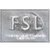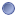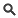### Module Detail Information

 Name: FSLMaths (nii.gz)Type:Module Short URL: `http://bit.ly/18aYTph` Description: Simple but powerful program to allow mathematical manipulation of images. Executable: `/fslmaths` Input Parameters: - Input File  - Add (image)  - Subtract (integer)  - Subtract (image)  - Multiply (integer)  - Multiply (image)  - Divide (integer)  - Divide (image)  - Mask  - Threshold  - Upper-Threshold  - Max (integer)  - Max (image)  - Min (integer)  - Min (image)  - Exponential  - Natural logarithm  - Square  - Square root  - Absolute Value  - Binarise  - Edge  - Replace NaN  - Make NaN  - X Mean  - Y Mean  - Z Mean  - T Mean  - X Std Dev  - Y Std Dev  - Z Std Dev  - T Std Dev  - X Max  - Y Max  - Z Max  - T Max  - X Max Index  - Y Max Index  - Z Max Index  - T Max Index  - X Min  - Z Min  - T Min  - X Median  - Y Median  - Z Median  - T Median  - X Percentage  - Y Percentage  - Z Percentage  - T Percentage  - X AR Coefficient  - Y AR Coefficient  - Z AR Coefficient  - T AR Coefficient  - Remainder (integer)  - Remainder (image)  - Threshold Percentage  - Threshold Percentage (non-zero voxels)  - Upper-Threshold Percentage  - Upper-Threshold Percentage (non-zero voxels)  - Index  - Grid  - TFCE  - Intensity Normalisation (per 3D volume mean)  - Intensity Normalisation (global 4D mean)  - Kernel 3D  - Kernel 2D  - Kernel Box  - Kernel BoxV  - Kernel Gauss  - Kernel Sphere  - Kernel File  - Mean Dilation  - Modal Dilation  - Maximum Filtering  - Erode  - Minimum Filtering  - Median Filtering  - Mean Filtering  - Mean Filtering (un-normalised)  - Sigma  - Downsample Image (centered)  - Downsample Image (non-centered)  - Region of Interest  - BPTF  - ROC  - 4D Noise Only  - AROC-thresh  - Truth  - Outfile  - Y Min  - Add (integer) Output Parameters: - Output File File size: 51.34 KBView SourceDownloadOpen